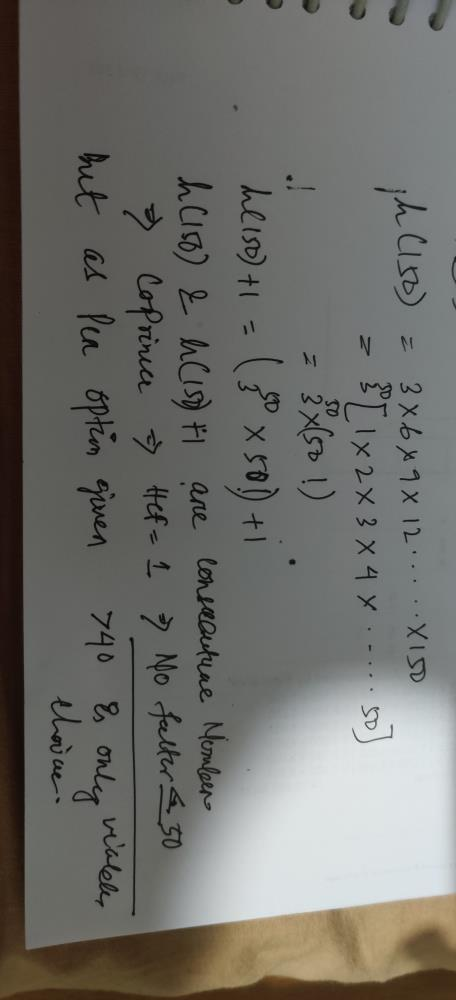Courses

# If h(x) = product of all the multiples of 3 from 3 to x, inclusive, then the smallest prime factor of h(150) + 1 must be………a)between 2 and 10b)between 11 and 20c)between 21 and 40d)greater than 40Correct answer is option 'D'. Can you explain this answer? Related Test: Test: Factors And Multiples- 1

## GMAT QuestionPreitee Ranjan Pradhan May 19, 2020This discussion on If h(x) = product of all the multiples of 3 from 3 to x, inclusive, then the smallest prime factor of h(150) + 1 must be………a)between 2 and 10b)between 11 and 20c)between 21 and 40d)greater than 40Correct answer is option 'D'. Can you explain this answer? is done on EduRev Study Group by GMAT Students. The Questions and Answers of If h(x) = product of all the multiples of 3 from 3 to x, inclusive, then the smallest prime factor of h(150) + 1 must be………a)between 2 and 10b)between 11 and 20c)between 21 and 40d)greater than 40Correct answer is option 'D'. Can you explain this answer? are solved by group of students and teacher of GMAT, which is also the largest student community of GMAT. If the answer is not available please wait for a while and a community member will probably answer this soon. You can study other questions, MCQs, videos and tests for GMAT on EduRev and even discuss your questions like If h(x) = product of all the multiples of 3 from 3 to x, inclusive, then the smallest prime factor of h(150) + 1 must be………a)between 2 and 10b)between 11 and 20c)between 21 and 40d)greater than 40Correct answer is option 'D'. Can you explain this answer? over here on EduRev! Apart from being the largest GMAT community, EduRev has the largest solved Question bank for GMAT.
This discussion on If h(x) = product of all the multiples of 3 from 3 to x, inclusive, then the smallest prime factor of h(150) + 1 must be………a)between 2 and 10b)between 11 and 20c)between 21 and 40d)greater than 40Correct answer is option 'D'. Can you explain this answer? is done on EduRev Study Group by GMAT Students. The Questions and Answers of If h(x) = product of all the multiples of 3 from 3 to x, inclusive, then the smallest prime factor of h(150) + 1 must be………a)between 2 and 10b)between 11 and 20c)between 21 and 40d)greater than 40Correct answer is option 'D'. Can you explain this answer? are solved by group of students and teacher of GMAT, which is also the largest student community of GMAT. If the answer is not available please wait for a while and a community member will probably answer this soon. You can study other questions, MCQs, videos and tests for GMAT on EduRev and even discuss your questions like If h(x) = product of all the multiples of 3 from 3 to x, inclusive, then the smallest prime factor of h(150) + 1 must be………a)between 2 and 10b)between 11 and 20c)between 21 and 40d)greater than 40Correct answer is option 'D'. Can you explain this answer? over here on EduRev! Apart from being the largest GMAT community, EduRev has the largest solved Question bank for GMAT.